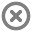Loading...## Info## Error# QuickMath

by Avantis US

Application ( 1MB )

Free

## Description

Quickmath is the Quick solution for all your math problems.
Solve quadratic, simultaneous, polynomial, find the roots, use general formula, find the angles of a right triangle, calculate the area, perimeter and volume of different geometrical shapes, and much more. This is a must have.
-Solve Simultaneous Equations up to 6 unknows.
Example:
X + Y + Z = 1
2X + 3Y - 4Z= 9
X - Y + Z = -1
The solution is x=1, y=1, z=-1
-Solve polynomial equations of degree 1 or degree 2 (Quadratic Equation).
Example:
X^2 -5X +6 =0
The solution is X1=3, X2=2
-It can find area, perimeter and volume of different geometric shapes including: triangle, right triangle, circle, square, ellipse, rectangle, trapezoid, pentagon, sphere, cube.
-Get the angles of the right triangle, and other figures
-Convert from different units, includes Number Base, Mass, Temperature, Distance, Volume.
Example:
Temperature 32celcius = 89.6 fahrenheit = 305.15 kelvin
Mass 253grams = 0.253kg = 8.924oz = 0.5577 lb